# CHSE Odisha Class 12 Math Solutions Chapter 8 Application of Derivatives Additional Exercise

Odisha State Board CHSE Odisha Class 12 Math Solutions Chapter 8 Application of Derivatives Additional Exercise Textbook Exercise Questions and Answers.

## CHSE Odisha Class 12 Math Solutions Chapter 8 Application of Derivatives Additional Exercise

(A) Multiple Choice Questions (Mcqs) With Answers

Question 1.
Write the maximum value of the function y = x5 in the interval [1, 5].
(a) 125
(b) 3125
(c) 625
(d) 225
Solution:
(b) 3125

Question 2.
Differentiate sin-1 (cos x) with respect to x.
(a) -1
(b) 0
(c) 1
(d) None of the above
Solution:
(a) -1

Question 3.
Write the equation of the tangent to the curve y = |x| at the point (-2, 2).
(a) 2x + 2y = 0
(b) 2x + y = 0
(c) x + y = 1
(d) x + y = 0
Solution:
(d) x + y = 0Question 4.
Write the value of $$\frac{d}{d x}$$(sin x)
(a) tan x
(b) sin x
(c) cos x
(d) cot x
Solution:
(c) cos x

Question 5.
Write the value of $$\frac{d}{d x}$$(cos x)
(a) cos x
(b) -sin x
(c) sin x
(d) cot x
Solution:
(b) -sin x

Question 6.
Write the value of $$\frac{d}{d x}$$(tan x)
(a) tan x
(b) sec2 x
(c) sin x
(d) cot x
Solution:
(c) sin x

Question 7.
Write the value of $$\frac{d}{d x}$$(sec x)
(a) sin x cos x
(b) sin x tan x
(c) cot x tan x
(d) sec x tan x
Solution:
(d) sec x tan x

Question 8.
Write the value of $$\frac{d}{d x}$$(cot x) = -cosec2x
(a) -sin2 x
(b) -cos2 x
(c) -cosec2 x
(d) -tan2 x
Solution:
(c) -cosec2 x

Question 9.
Write the value of $$\frac{d}{d x}$$(cosec x)
(a) -cosec x cot x
(b) -sin x cot x
(c) -tan x cot x
(d) -cos x cot x
Solution:
(a) -cosec x cot x

Question 10.
Write the value of $$\frac{d}{d x}$$(ex) = ex
(a) e
(b) x
(c) ex
(d) e2x
Solution:
(c) ex

Question 11.
Write the value of $$\frac{d}{d x}$$(ax)
(a) a2x log a
(b) ax log a
(c) log a
(d) log ax
Solution:
(b) ax log a

Question 12.
Write the value of $$\frac{d}{d x}$$(log x)
(a) x
(b) $$\frac{1}{2 x}$$
(c) $$\frac{1}{x^2}$$
(d) $$\frac{1}{x}$$
Solution:
(d) $$\frac{1}{x}$$

Question 13.
Write the value of $$\frac{d}{d x}$$(loga x)
(a) $$\frac{x}{\log a}$$
(b) $$\frac{1}{x \log a}$$
(c) $$\frac{1}{x^2 \log a}$$
(d) $$\frac{1}{2 x^2 \log a}$$
Solution:
(b) $$\frac{1}{x \log a}$$

Question 14.
Write the value of $$\frac{d}{d x}$$(cot-1 x) = $$\frac{-1}{1+x^2}$$
(a) $$\frac{1}{1-x^2}$$
(b) $$\frac{1}{1+x^2}$$
(c) $$\frac{-1}{1-x^2}$$
(d) $$\frac{-1}{1+x^2}$$
Solution:
(d) $$\frac{-1}{1+x^2}$$

Question 15.
Find $$\frac{\mathrm{dy}}{\mathrm{dx}}$$ for √x + √y = √c.
(a) $$\frac{\sqrt{y}}{\sqrt{x}}$$
(b) $$\frac{\sqrt{x}}{\sqrt{y}}$$
(c) –$$\frac{\sqrt{y}}{\sqrt{x}}$$
(d) –$$\frac{\sqrt{x}}{\sqrt{y}}$$
Solution:
(c) –$$\frac{\sqrt{y}}{\sqrt{x}}$$

Question 16.
If f(x) = $$\log _{x^2}$$ (log x), then find f'(e).
(a) $$\frac{1}{e}$$
(b) $$\frac{1}{\mathrm{e}^2}$$
(c) $$\frac{1}{2 \mathrm{e}}$$
(d) $$\frac{2}{\mathrm{e}}$$
Solution:
(c) $$\frac{1}{2 \mathrm{e}}$$Question 17.
If y = $$\sqrt{\sin x+y}$$, then what is $$\frac{\mathrm{dy}}{\mathrm{dx}}$$?
(a) $$\frac{\cos x}{2 y+1}$$
(b) $$\frac{\cos x}{2 y-1}$$
(c) $$\frac{\sin x}{2 y-1}$$
(d) $$\frac{\sin x}{2 y+1}$$
Solution:
(b) $$\frac{\cos x}{2 y-1}$$

Question 18.
If y = 10x and z = 100x/2 then find $$\frac{\mathrm{d}}{\mathrm{dx}}\left(\frac{\mathrm{y}^2}{\mathrm{z}}\right)$$
(a) 10x log 10
(b) 102x log 10
(c) x log 10
(d) x2 log 10
Solution:
(a) 10x log 10

Question 19.
Find the differential of y = sin2 x.
(a) 2sinx cot x dx
(b) 2sinx tan x dx
(c) 2sinx cosx dx
(d) 2cosx sinx dx
Solution:
(c) 2sinx cosx dx

Question 20.
Differentiate $$\sqrt{\sin \sqrt{x}}$$
(a) $$\frac{\cos \sqrt{x}}{4 \sqrt{x \sin \sqrt{x}}}$$
(b) $$\frac{\sin x}{4 \sqrt{x \cos \sqrt{x}}}$$
(c) $$\frac{\sin \sqrt{x}}{4 \sqrt{x \cos \sqrt{x}}}$$
(d) $$\frac{\sin x}{4 \sqrt{x \cos x}}$$
Solution:
(a) $$\frac{\cos \sqrt{x}}{4 \sqrt{x \sin \sqrt{x}}}$$

Question 21.
If f(x) = [tan2 x], what is f'(0)?
(a) 0
(b) 1
(c) -1
(d) None of the above
Solution:
(a) 0

Question 22.
If xy = ex-y then find $$\frac{\mathrm{dy}}{\mathrm{dx}}$$.
(a) $$\frac{\log x}{(1-\log x)^2}$$
(b) $$\frac{\log x}{(1+\log x)^2}$$
(c) $$\frac{\log x}{(1+\log x)}$$
(d) $$\frac{\log x}{(1-\log x)}$$
Solution:
(b) $$\frac{\log x}{(1+\log x)^2}$$

Question 23.
Find $$\frac{\mathrm{dy}}{\mathrm{dx}}$$ if y = cot-1$$\left(\frac{1+\cos x}{1-\cos x}\right)^{\frac{1}{2}}$$
(a) 0
(b) $$\frac{1}{2}$$
(c) 1
(d) $$\frac{1}{4}$$
Solution:
(b) $$\frac{1}{2}$$

Question 24.
Find the derivative of sin x° w.r.t. x.
(a) $$\frac{\pi}{90}$$ cos x°
(b) π cos x°
(c) $$\frac{\pi}{180}$$ cos x°
(d) π sin x°
Solution:
(c) $$\frac{\pi}{180}$$ cos x°

Question 25.
The least value of a such that f(x) = x2 + ax + 1 is strictly increasing on (1, 2) is
(a) -2
(b) -4
(c) 2
(d) 4
Solution:
(a) -2

Question 26.
If y = sec-1$$\left(\frac{x+1}{x-1}\right)$$ + sin-1$$\left(\frac{x-1}{x+1}\right)$$ then find $$\frac{\mathrm{dy}}{\mathrm{dx}}$$.
(a) 0
(b) -1
(c) 1
(d) None of the above
Solution:
(a) 0Question 27.
Let f(x) = ex g(x), g(0) = 2 and g'(0) = 1, then find f'(0).
(a) 1
(b) 2
(c) 3
(d) 0
Solution:
(c) 3

Question 28.
If x ∈ (0, π/2) then find $$\frac{\mathrm{d}}{\mathrm{dx}}$$[sin x]
(a) 0
(b) 1
(c) 7C
(d) $$\frac{\pi}{2}$$
Solution:
(a) 0

Question 29.
If f(x) = [x2] then f’$$\left(\frac{3}{2}\right)$$ = ________.
(a) 1
(b) $$\frac{3}{2}$$
(c) 0
(d) –$$\frac{3}{2}$$
Solution:
(c) 0

Question 30.
A differentiable function f defined for all x > 0 satisfies f(x2) = x3 for all x > 0. What is f'(b)?
(a) 3
(b) 4
(c) 6
(d) 12
Solution:
(c) 6

Question 31.
What is the derivative of f'(In x) w.r.t x where f(x) = ln x.
(a) $$\frac{1}{x^2 \ln x}$$
(b) $$\frac{1}{2 x \ln x}$$
(c) $$\frac{1}{x \ln x^2}$$
(d) $$\frac{1}{x \ln x}$$
Solution:
(d) $$\frac{1}{x \ln x}$$

Question 32.
For what value of a, f(x) = loga (x) is increasing on R?
(a) a > 1
(c) a < 1
(b) a = 1
(d) a > 1
Solution:
(a) a ≥ 1

Question 33.
Write the points at which tangent to the curve y = x2 – 3x is parallel to x – axis.
(a) $$\left(\frac{3}{2}, \frac{9}{4}\right)$$
(b) $$\left(\frac{3}{2}, \frac{-9}{4}\right)$$
(c) $$\left(\frac{-3}{2}, \frac{9}{4}\right)$$
(d) $$\left(\frac{-3}{2}, \frac{-9}{4}\right)$$
Solution:
(b) $$\left(\frac{3}{2}, \frac{-9}{4}\right)$$

Question 34.
If y = ex + e-x + 2 has a tangent parallel to x – axis at (α, β) then find the value of α.
(a) 0
(b) 2
(c) 1
(d) -1
Solution:
(a) 0

Question 35.
Write slope of the tangent to the curve y = √3 sin x + cos x at ($$\frac{\pi}{3}$$, 2)
(a) 1
(b) -1
(c) 0
(d) None of the above
Solution:
(c) 0Question 36.
Find the value of x for which f(x) is either a local maximum or a local minimum when
f(x) = x3 – 3x2 – 9x + 6.
(a) (3, -1)
(b) (3, 1)
(c) (-1, 3)
(d) (1, -3)
Solution:
(a) (3, -1)

Question 37.
Find the x – coordinates of the extreme points of the function.
y = cos x + sin x , x ∈ [0, $$\frac{\pi}{2}$$]
(a) $$\frac{\pi}{4}$$
(b) $$\frac{2 \pi}{3}$$
(c) $$\frac{\pi}{2}$$
(d) π
Solution:
(a) $$\frac{\pi}{4}$$

Question 38.
Find the equation of tangent to the curve x = y2 – 2 at the points where slope of the normal equal to (-2).
(a) x + y – 1 = 0
(b) 2x + y – 1 = 0
(c) 2x – y + 1 = 0
(d) x – y + 1 = 0
Solution:
(b) 2x + y – 1 = 0

Question 39.
f(x) = x4 – 62x2 + ax + 9 attains its maximum value at x = 1 in the interval [0, 2]. Find the value of ‘a’.
(a) 12
(b) 120
(c) 1
(d) 1200
Solution:
(b) 120

Question 40.
If sin (x + y) = log (x + y) then $$\frac{d y}{d x}$$ is:
(a) 2
(b) -2
(c) 1
(d) -1
Solution:
(d) -1

Question 41.
If f(x) = 2x and g(x) = $$\frac{x^2+1}{2}$$ then which of the following can be a discontinuous function?
(a) F(x) + g(x)
(b) f(x) – g(x)
(c) f(x) . g(x)
(d) $$\frac{f(x)}{g(x)}$$
Solution:
(d) $$\frac{f(x)}{g(x)}$$

Question 42.
If f(x) = x2 sin (‘) where x ≠ 0 then the value of the function f at x = 0, so that the function is continuous at x = 0 is
(a) 0
(b) -1
(c) 1
(d) none of these
Solution:
(a) 0

Question 43.
The derivative of cos-1 (2x2 – 1) with respect to cos-1 x
(a) 2
(b) $$\frac{-1}{2 \sqrt{1-x^2}}$$
(c) $$\frac{2}{x}$$
(d) 1 – x2
Solution:
(a) 2

Question 44.
If y = $$\sqrt{\sin x+y}$$ then $$\frac{d y}{d x}$$ is equal to:
(a) $$\frac{\cos x}{2 y-1}$$
(b) $$\frac{\cos x}{1-2 y}$$
(c) $$\frac{\sin x}{1-2 y}$$
(d) $$\frac{\sin x}{2 y-1}$$
Solution:
(a) $$\frac{\cos x}{2 y-1}$$Question 45.
Find the value of $$\frac{d y}{d x}$$ at θ = $$\frac{\pi}{3}$$ if x = asec3 θ and x = atan3 θ is:
(a) $$\frac{\sqrt{3}}{2}$$
(b) –$$\frac{\sqrt{3}}{2}$$
(c) $$\frac{1}{2}$$
(d) 1
Solution:
(a) $$\frac{\sqrt{3}}{2}$$

Question 46.
If x2y = ex-y then $$\frac{d y}{d x}$$ is:
(a) $$\frac{1+x}{1+\log x}$$
(b) $$\frac{1-x}{1+\log x}$$
(c) $$\frac{x}{1+\log x}$$
(d) $$\frac{x}{(1+\log x)^2}$$
Solution:
(d) $$\frac{x}{(1+\log x)^2}$$

Question 47.
Differential coefficient of sec (tan-1 x) is:
(a) $$\frac{x}{1+x^2}$$
(b) $$x \sqrt{1+x^2}$$
(c) $$\frac{x}{\sqrt{1+x^2}}$$
(d) $$\frac{1}{\sqrt{1+x^2}}$$
Solution:
(c) $$\frac{x}{\sqrt{1+x^2}}$$

Question 48.
The function f(x) = xx is decreasing in the interval:
(a) (0, e)
(b) (0, $$\frac{1}{e}$$)
(c) (0, 1)
(d) none of these
Solution:
(b) (0, $$\frac{1}{e}$$)

(B) Very Short Type Questions With Answers

Question 1.
If f'(2+) = 0 and f'(2) = 0, then is f(x) continuous at x = 2?
Solution:
f'(2+) = 0 and f'(2) = 0
⇒ f is differentiable at x = 2
⇒ f is continuous at x = 2.

Question 2.
If Φ(x) = f(x) + f(1 – x), f'(x) = 0 for 0 < x < 1, then is x = a point of maxima or minima of Φ(x)?
Solution:
Let Φ(x) = f(x) + f(1 – x)
⇒ Φ’ = f'(x) – f'(1 – x)
Φ”(x) = f”(x) + f”(1 – x)
Now Φ'($$\frac{1}{2}$$) = f'($$\frac{1}{2}$$) – f'($$\frac{1}{2}$$) = 0
⇒ $$\frac{1}{2}$$ is a critical point.
Φ”($$\frac{1}{2}$$) = f”($$\frac{1}{2}$$) – f”($$\frac{1}{2}$$) = 0
⇒ x = $$\frac{1}{2}$$ is neither a point of local maxima nor a point of local minima.

Question 3.
Write the interval in which the function f(x) = sin-1 (2 – x) is differentiable.
Solution:
f(x) = sin-1 (2 – x) is differentiable for
1 – (2 – x)2 > 0.
⇒ (2 – x)2 < 1
⇒ -1 < 2 – x < 1
⇒ -3 < -x < -1
⇒ 3 > x < 1
⇒ 1 < x < 3
⇒ x ∈ (1, 3)
∴ f is differentiable on (1, 3)Question 4.
Write the set of values of x for which the function f(x) = sin x – x is increasing.
Solution:
f(x) = sin x – x
⇒ f'(x) = cos x – 1 ≤ 0 for all x ∈ R
∴ f(x) is increasing for all x ∈ R

Question 5.
Write the differential coefficient of e[x] ln (x + 1) where 3 ≤ x < 4 with respect to x.
Solution:
Let y = e
= e3ln(x+1) (3 ≤ x < 4)
= $$e^{\ln (x+1)^3}$$ = (x + 1)3
$$\frac{d y}{d x}$$ = 3(x + 1)2

Question 6.
Write a logarithmic function which is differentiable only in the open interval (-1, 1).
Solution:
f(x) = $$\left\{\begin{array}{l} \ln \left|\sin ^{-1} x\right|, x \neq 0 \\ 1, x=0 \end{array}\right.$$ is differentiable only on (-1, 1).

Question 7.
Write a function which has both relative and absolute maximum at the point (1, 2).
Solution:
f(x) = -x2 + 2x + 1 on [0, 1] has relative and absolute maximum at (1, 2).

Question 8.
Write the derivation of e3logx with respect to x2.
Solution:
Let u = e3lnx = elnx = $$e^{\ln x^3}$$= x3 and v = x2
∴ $$\frac{d u}{d x}$$ = 3x2 and $$\frac{d v}{d x}$$ = 2x
∴ Derivative of u w.r.t. v is
$$\frac{d u}{d v}$$ = $$\frac{3 x^2}{2 x}$$ = $$\frac{3 x}{2}$$

Question 9.
Write the maximum value of the function y = x5 in the interval [1, 5]:
Solution:
y = x5
⇒ $$\frac{d y}{d x}$$ = 5x4 > 0 ∀ x ∈ [1, 5]
i.e y is strictly increasing in the given interval.
Thus the maximum value of
y = x5 = 55 = 3125

Question 10.
Differentiate alnx with respect to x
Solution:
$$\frac{d}{d x}$$(aln x) = aln x (ln a)$$\frac{d}{d x}$$ln x
= $$\frac{a^{\ln x} \ln a}{x}$$Question 11.
Mention the values of x for which x the function f(x) = x3 – 12x is increasing.
Solution:
Given f(x) = x3 – 12x
f is increasing if f'(x) > 0
⇒ 3x2 – 12 > 0
⇒ x2 > 4
⇒ -2 > x > 2
⇒ x ∈ (-∞, -2) ∪ (2, ∞)

Question 12.
Differentiate sin-1 (cos x) with respect to x.
Solution:
$$\frac{d}{d x}$$sin-1 (cos x) = $$\frac{d}{d x}$$sin-1 sin ($$\frac{\pi}{2}$$ – x)
= $$\frac{d}{d x}$$ ($$\frac{\pi}{2}$$ – x) = 1

Question 13.
Write the equation of the tangent to the curve y = |x| at the point (-2, 2).
Solution:
y = |x| = \left\{\begin{aligned} x, & x \geq 0 \\ -x, & x<0 \end{aligned}\right.
When x < 0, $$\frac{d y}{d x}$$ = -1
which is the slope of the tangent at (-2, 2).
Equation of the required tangent is
y – 2 = (-1) (x + 2)
⇒ y = -x
⇒ x + y = 0.

Question 14.
What is the derivative of sec-1 x with respect to x if x < -1?
Solution:
Let y = sec-1 x
⇒ $$\frac{d y}{d x}$$ = $$\frac{1}{|x| \sqrt{x^2-1}}$$
when x < (-1) we have |x| = -x
∴ $$\left.\frac{\mathrm{dy}}{\mathrm{dx}}\right]_{\mathrm{x}<(-1)}$$ = $$\frac{(-1)}{x \sqrt{x^2-1}}$$

Question 15.
Write the set of points where the function f(x) = x3 has relative (local) extrema.
Solution:
f(x) = x3
⇒ f'(x) = 3x2
f'(x) = 0
⇒ x = 0
But f”(x) = 6x = 0 when x = 0
∴ x = 0 is not a point of local extrema.
∴ Hence the set of points where f has relative extrema = Φ.

Question 16.
In which interval of x the function $$\frac{\ln \mathbf{x}}{x}$$ is decreasing?
Solution:
Let f(x) = $$\frac{\ln x}{x}$$
⇒ f'(x) = $$\frac{x \cdot \frac{1}{x}-\ln x}{x^2}$$ = $$\frac{1-\ln x}{x^2}$$
f is decreasing for
f'(x) < 0 ⇒ $$\frac{1-\ln x}{x^2}$$ < 0
⇒ 1 – ln x < 0 ( x2 > 0)
⇒ 1 < ln x
⇒ ln x > 1
⇒ x e (e, ∞).(C) Short Type Questions With Answers

Question 1.
If y = $$e^{x^{e^{e^{e^{x^x…..}}}}}$$, then find $$\frac{d \mathbf{y}}{\mathbf{d x}}$$.
Solution: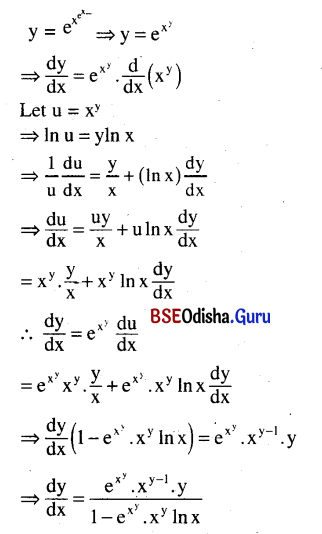Question 2.
If $$\frac{\mathbf{d}^2 \mathbf{y}}{\mathbf{d x}^2}$$, if x = a cosΦ and y = b sinΦ.
Solution:
x = a cosΦ, y = b sinΦ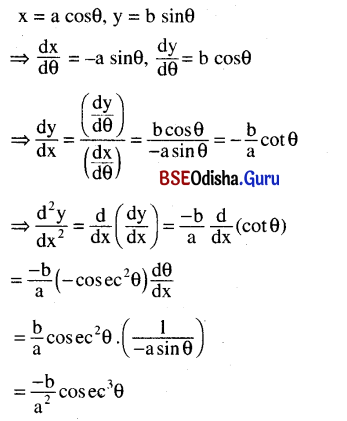Question 3.
Find the point on the curve x2 + y2 – 4xy + 2 = 0, where the normal to the curve is parallel to the x – axis.
Solution:
Given equation of the curve is
x2 + y2 – 4xy + 2 = 0 … (1)
Differentiang we getQuestion 4.
Find the intervals in which the function y = $$\frac{\ln x}{x}$$ is increasing and decreasing.
Solution: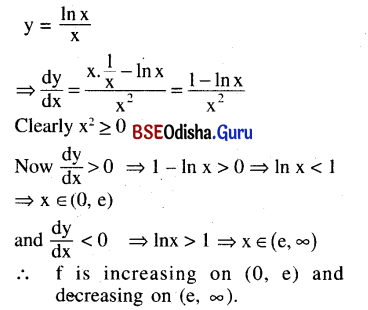Question 5.
Differentiate y = tan-1$$\frac{\sqrt{1+x^2}+\sqrt{1-x^2}}{\sqrt{1+x^2}-\sqrt{1-x^2}}$$
Solution: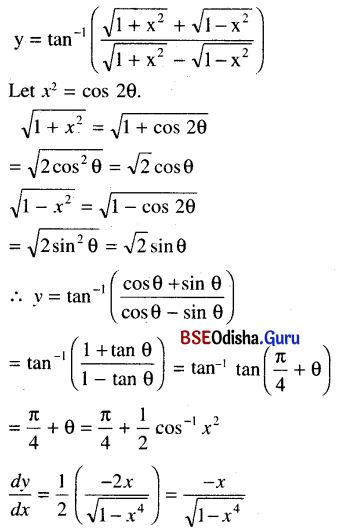Question 6.
Differentiate y = (sin y)sin 2x
Solution:
y = (sin y)sin 2x
⇒ In y = sin 2x ln (sin y)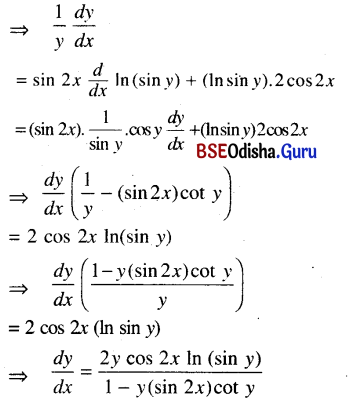Question 7.
Test the differentiability and continuity of the following function at x = 0:
f(x) = $$\left\{\begin{array}{cc} \frac{1-e^{-x}}{x} & x \neq 0 \\ 1 & x=0 \end{array}\right.$$
Solution:
Given function is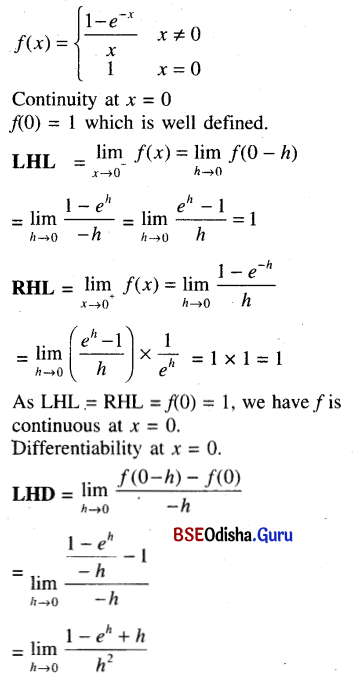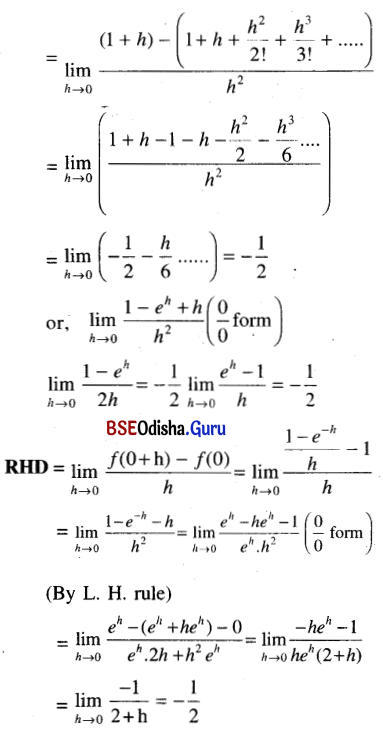As LHD = RHD, we have the given function is differentiable at x = 0 and f'(0) = –$$\frac{1}{2}$$.

Question 8.
Show that the sum of intercepts on the co-ordinate axes of any tangent to the curve
√x + √y = √a is constant.
Solution:
Given curve √x + √y = √a … (1)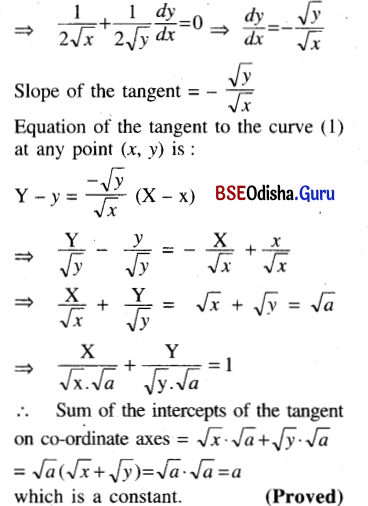Question 9.
Find the equation of the normal to the curve y = (log x)2 at x = $$\frac{1}{e}$$.
Solution:
Given equation of the curve is
y = (log x)2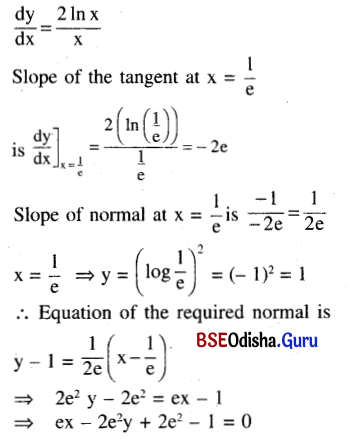Question 10.
If y = x + $$\frac{1}{x+\frac{1}{x+\cdots \cdots \infty}}$$, find $$\frac{\mathbf{d y}}{\mathbf{d x}}$$, the rhs being a valid expression.
Solution: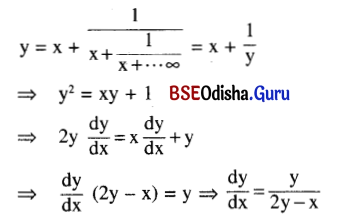Question 11.
Differentiate sec-1 ($$\frac{1}{2 x^2-1}$$) with respect to $$\sqrt{1-x^2}$$.
Solution: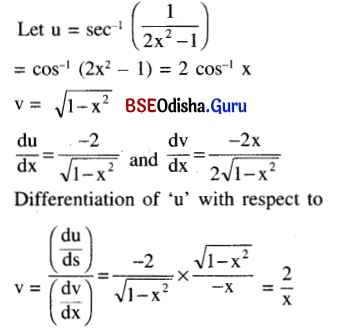Question 12.
Find $$\frac{d \mathbf{y}}{\mathbf{d t}}$$ , when y = sin-1 $$\left(\frac{2 \sqrt{t}-1}{t^2}\right)$$
Solution: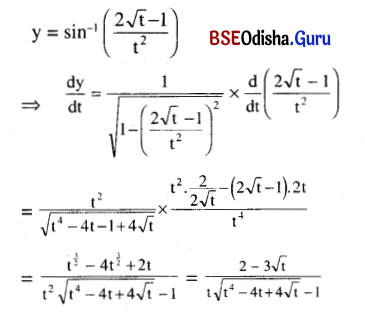Question 13.
Find $$\frac{\mathbf{d y}}{\mathbf{d x}}$$ , if xmyn = $$\left(\frac{\mathbf{x}}{\mathbf{y}}\right)^{\mathrm{m}+\mathrm{n}}$$
Solution: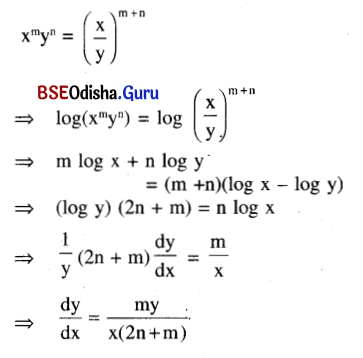Question 14.
If x = a sec θ, y = b tan θ, prove that:
$$\frac{d^2 y}{d x^2}$$ = –$$\frac{b^4}{a^2 y^3}$$
Solution: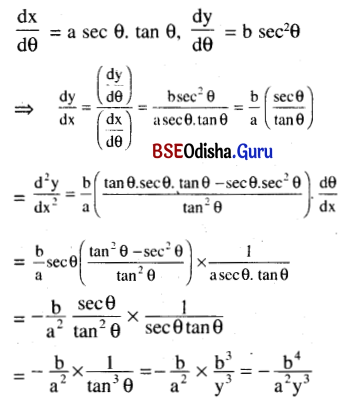Question 15.
Find the interval where the following function is increasing:
y = sin x + cos x, x ∈ [0, 2π].
Solution:
y = sin x + cos x, x ∈ [0, 2π].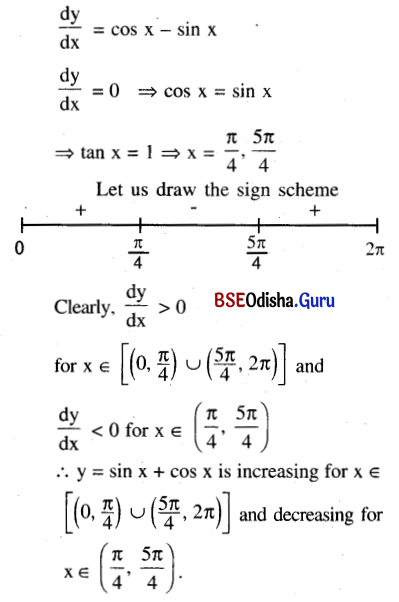Question 16.
Find $$\frac{d y}{d x}$$, when yx = xsin y.
Solution:
yx = xsin y
Taking log of both sides we get,
x ln y = (sin y) ln x
Differentiating with respect to x we get,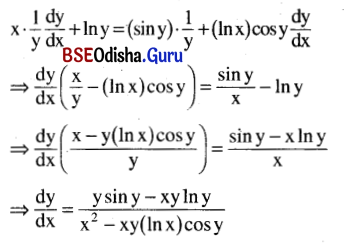Question 17.
Show that $$\frac{d y}{d x}$$ is independent of t,
if cos x = $$\sqrt{\frac{1}{1+t^2}}$$, sin y = $$\frac{2 t}{1+t^2}$$
Solution:
cos x = $$\sqrt{\frac{1}{1+t^2}}$$, sin y = $$\frac{2 t}{1+t^2}$$
Let t = tan θ
∴ cos x = cos θ, sin y = sin 2θ
⇒ y = 2θ, x = θ
⇒ y = 2x
⇒ $$\frac{d y}{d x}$$ = 2 which is independent of t.

Question 18.
Show that 2sin x + tan x > 3x for all x ∈ (0, $$\frac{\pi}{2}$$)
Solution:
Let f(x) = 2sin x + tan x – 3x
⇒ f'(x) = 2cos x + sec2 x – 3
Let g(x) = f'(x) = 2cos x + sec2 x – 3
⇒ g'(x) = -2sin x + 2sec2 x tan x
= 2sin x (sec3 x – 1) ≥ 0
for all x ∈ (0, $$\frac{\pi}{2}$$)
∴ g is an increasing function on (0, $$\frac{\pi}{2}$$)
But g(0) = 0
∴ g(x) ≥ 0 for all x ∈ (0, $$\frac{\pi}{2}$$)
⇒ f(x) ≥ 0 for all x ∈ (0, $$\frac{\pi}{2}$$)
⇒ f is an increasing function on (0, $$\frac{\pi}{2}$$)
But f(0) = 0
∴ f(x) ≥ 0 for all x ∈ (0, $$\frac{\pi}{2}$$)
⇒ 2sin x + tan x ≥ 3x for x ∈ (0, $$\frac{\pi}{2}$$)Question 19.
Show that no two normals to a parabola are parallel.
Solution:
Let us consider the parabola y2 = 4ax – (1) and A(at12, 2at1) and B (at22, 2at2) are any two points on its from (1)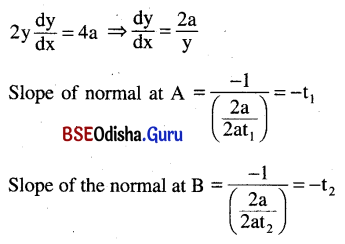As t1 ≠ t2 we have no two normals of a parabola are parallel.

Question 20.
Examine the differentiability of In x2 for all real values of x.
Solution:
Let f(x) = In (x2) = 2 ln |x|
Clearly Dom f = R – {0}
Let any a ∈ Dom f.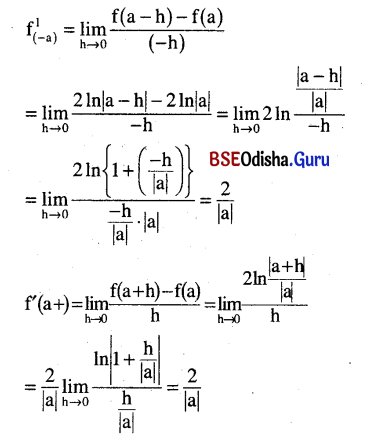Question 21.
Find the derivative of xsin x with respect to x.
Solution:
Let y = xsin x = e(sin x)/nx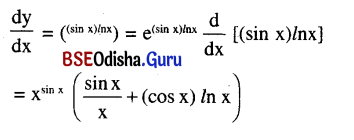Question 22.
Differentiate sin-1 $$\left(\frac{2 x}{1+x^2}\right)$$ with respect to cos-1 $$\left(\frac{1-x^2}{1+x^2}\right)$$.
Solution: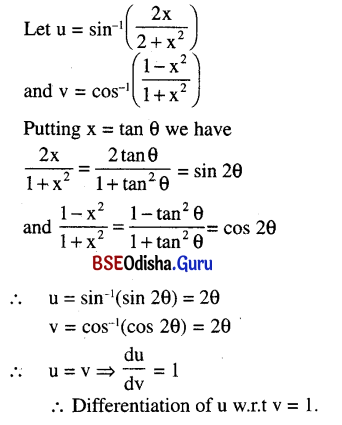Question 23.
Find the slope of the tangent to the curve x = 2(t – sin t), y = 2(1 – cos t) at t = $$\frac{\pi}{4}$$.
Solution: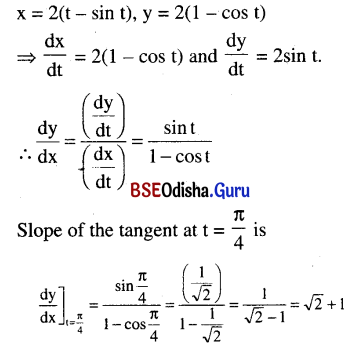Question 24.
If cos y = x cos(a + y), then show that $$\frac{d y}{d x}$$ = $$\frac{\cos ^2(a+y)}{\sin a}$$.
Solution: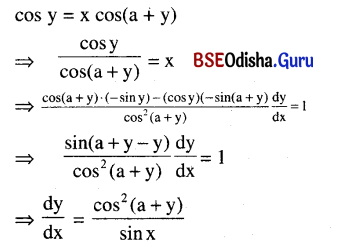Question 25.
Find the extreme values of the function y = x + $$\frac{y}{x}$$.
Solution: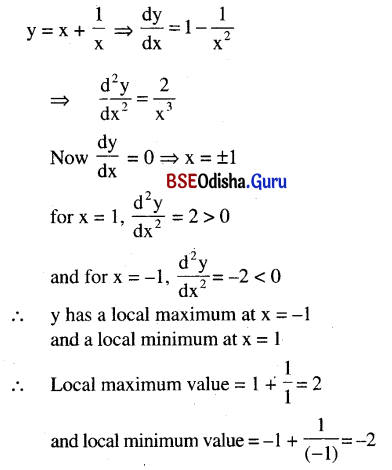Question 26.
Find the intervals where the following function is (i) increasing, (ii) decreasing:
f(x) = $$\begin{cases}\mathbf{x}^2+1, & x \leq-3 \\ x^3-8 x+13, & x>-3\end{cases}$$
Solution:
Given f(x) = $$\begin{cases}\mathbf{x}^2+1, & x \leq-3 \\ x^3-8 x+13, & x>-3\end{cases}$$
Clearly f is not differentiable at x = -3.
and f ‘(x) = $$\begin{cases}2 \mathrm{x}, & \mathrm{x}>-3 \\ 3 \mathrm{x}^2-8 & \mathrm{x}<-3 \\ \text { does not exist } & \text { for } \mathrm{x}=-3\end{cases}$$
Case – 1: x > -3
Clearly f'(x) > 0 for x > 0 and f'(x) < 0 for -3 < x < 0
∴ f is increasing in (0, ∞) and decreasing in (-3, 0).
Case – 2: x < -3
Clearly for x < -3, f(x) = 3x2 – 8 > 0
i.e., f is increasing in (-∞, -3)
Thus f is increasing in (-∞, -3) ∪ (0, ∞) and decreasing in (-3, 0).Question 27.
Prove that:
y = In tan $$\left(\frac{\pi}{4}+\frac{x}{2}\right)$$ ⇒ $$\frac{d y}{d x}$$ = sec x
Solution: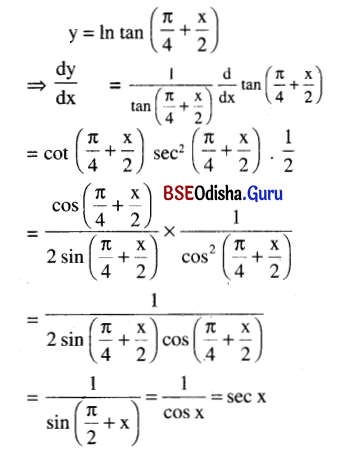Question 28.
Differentiate with respect to x:
y = $$2^{x^2}$$ + tan-1 $$\left(\frac{\cos x-\sin x}{\cos x+\sin x}\right)$$
Solution: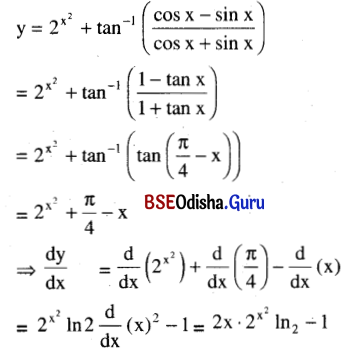Question 29.
Find the equation of the tangent to the curve x = y2 – 1 at the point where the slope of the normal to the curve is (-2).
Solution:
Given equation of the curve is x = y2 – 1.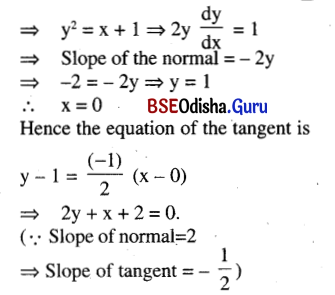Question 30.
Find $$\frac{d y}{d x}$$ if y = $$\log _{\left(x^2\right)}$$3.
Solution: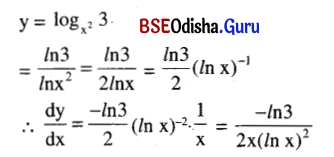Question 31.
Write why the function sin-1 $$\frac{1}{\sqrt{1-x^2}}$$ cannot be differentiated anywhere.
Solution: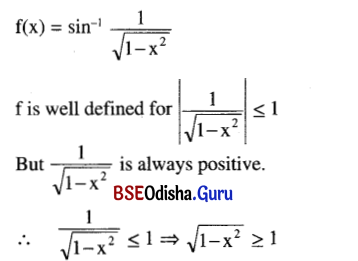⇒ 1 – x2 ≥ 1
⇒ x = 0
∴ DOM of {0 3}
But f is not defined in a deleted interval of x = 0.
Hence f is not differentiable at x = 0 and hence not differentiable anywhere.